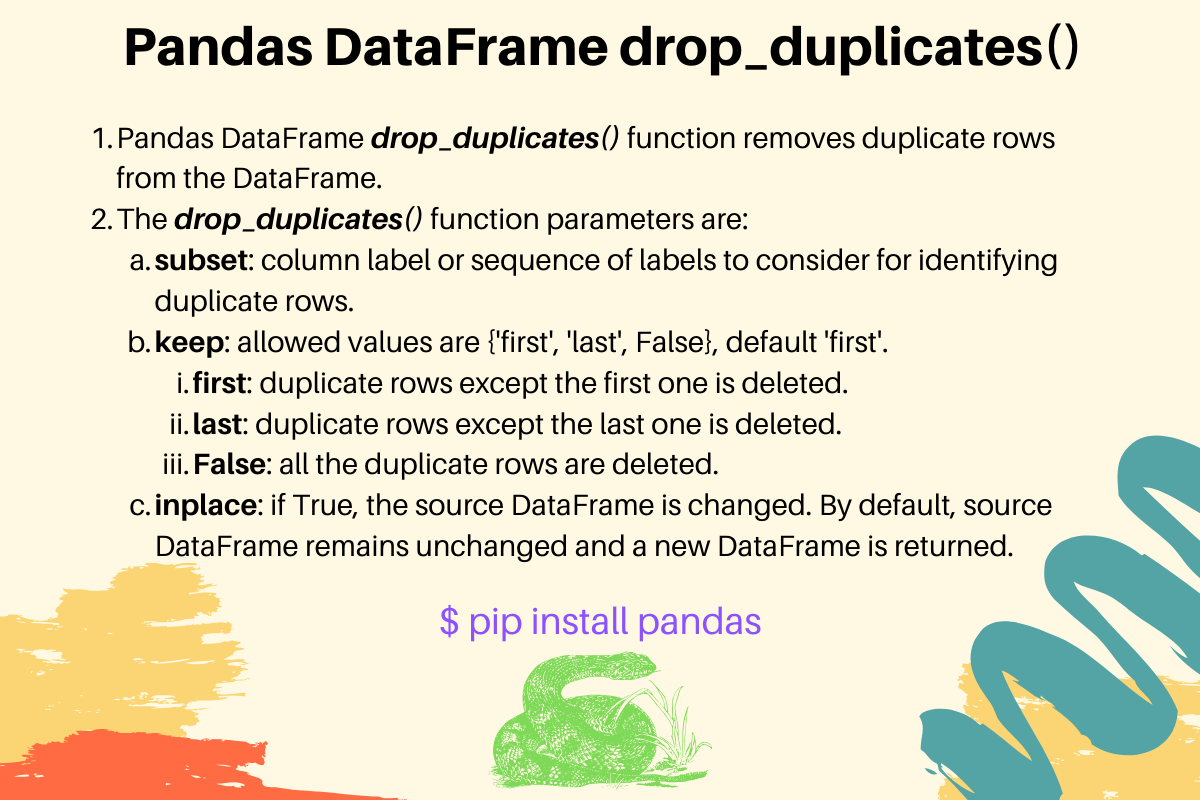# Pandas Drop Duplicate Rows - drop_duplicates() function

Published on August 3, 2022By Pankaj
Developer and author at DigitalOcean.While we believe that this content benefits our community, we have not yet thoroughly reviewed it. If you have any suggestions for improvements, please let us know by clicking the “report an issue“ button at the bottom of the tutorial.

## Pandas drop_duplicates() Function Syntax

Pandas drop_duplicates() function removes duplicate rows from the DataFrame. Its syntax is:

``````drop_duplicates(self, subset=None, keep="first", inplace=False)
``````
• subset: column label or sequence of labels to consider for identifying duplicate rows. By default, all the columns are used to find the duplicate rows.
• keep: allowed values are {‘first’, ‘last’, False}, default ‘first’. If ‘first’, duplicate rows except the first one is deleted. If ‘last’, duplicate rows except the last one is deleted. If False, all the duplicate rows are deleted.
• inplace: if True, the source DataFrame is changed and None is returned. By default, source DataFrame remains unchanged and a new DataFrame instance is returned.

## Pandas Drop Duplicate Rows Examples

Let’s look into some examples of dropping duplicate rows from a DataFrame object.

### 1. Drop Duplicate Rows Keeping the First One

This is the default behavior when no arguments are passed.

``````import pandas as pd

d1 = {'A': [1, 1, 1, 2], 'B': [2, 2, 2, 3], 'C': [3, 3, 4, 5]}

source_df = pd.DataFrame(d1)
print('Source DataFrame:\n', source_df)

# keep first duplicate row
result_df = source_df.drop_duplicates()
print('Result DataFrame:\n', result_df)
``````

Output:

``````Source DataFrame:
A  B  C
0  1  2  3
1  1  2  3
2  1  2  4
3  2  3  5
Result DataFrame:
A  B  C
0  1  2  3
2  1  2  4
3  2  3  5
``````

The source DataFrame rows 0 and 1 are duplicates. The first occurrence is kept and the rest of the duplicates are deleted.

### 2. Drop Duplicates and Keep Last Row

``````result_df = source_df.drop_duplicates(keep='last')
print('Result DataFrame:\n', result_df)
``````

Output:

``````Result DataFrame:
A  B  C
1  1  2  3
2  1  2  4
3  2  3  5
``````

The index ‘0’ is deleted and the last duplicate row ‘1’ is kept in the output.

### 3. Delete All Duplicate Rows from DataFrame

``````result_df = source_df.drop_duplicates(keep=False)
print('Result DataFrame:\n', result_df)
``````

Output:

``````Result DataFrame:
A  B  C
2  1  2  4
3  2  3  5
``````

Both the duplicate rows ‘0’ and ‘1’ are dropped from the result DataFrame.

### 4. Identify Duplicate Rows based on Specific Columns

``````import pandas as pd

d1 = {'A': [1, 1, 1, 2], 'B': [2, 2, 2, 3], 'C': [3, 3, 4, 5]}

source_df = pd.DataFrame(d1)
print('Source DataFrame:\n', source_df)

result_df = source_df.drop_duplicates(subset=['A', 'B'])
print('Result DataFrame:\n', result_df)
``````

Output:

``````Source DataFrame:
A  B  C
0  1  2  3
1  1  2  3
2  1  2  4
3  2  3  5
Result DataFrame:
A  B  C
0  1  2  3
3  2  3  5
``````

The columns ‘A’ and ‘B’ are used to identify duplicate rows. Hence, rows 0, 1, and 2 are duplicates. So, rows 1 and 2 are removed from the output.

### 5. Remove Duplicate Rows in place

``````source_df.drop_duplicates(inplace=True)
print(source_df)
``````

Output:

``````   A  B  C
0  1  2  3
2  1  2  4
3  2  3  5
``````